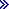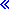%CHAR(numeric)%CHAR can convert the value of a numeric expression to type character.If the value of the expression is float, the result will be in float format (for example '+1.125000000000000E+020'). Otherwise, the result will be in decimal format with a leading negative sign if the value is negative, and without leading zeros. The character used for any decimal point will be the character indicated by the control specification DECEDIT keyword (default is '.'). For example, %CHAR of a packed(7,3) expression might return the value '-1.234'.The CCSID of the returned value is *JOBRUN.For more information, see Conversion Operations or Built-in Functions.%CHAR Examples with numeric parameters

D result          S            100A   VARYING
D points          S             10I 0 INZ(234)
D total           S              7P 2 INZ(523.45)
D adjust          S              7P 2 INZ(-123.45)
D variance        S              8F   INZ(.01234)
This example converts an integer value to character format:
result = 'You have ' + %char(points) + ' points.';
// result = 'You have 234 points.'
This example converts a float value to character format:
result = 'The variance is ' + %char(variance);
// result = 'The variance is +1,234000000000000E-002'
This example converts packed values to character format:
result = 'Total is ' + %char(total) + ' and '